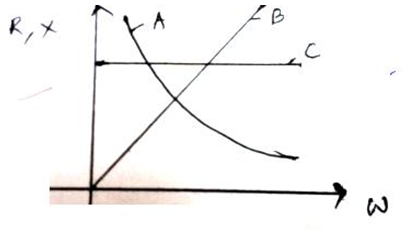# NEET Physics Alternating Current Questions Solved

(a) Figure shows the variation of resistance and reactance versus angular frequency. Identify the curve which corresponds to inductive reactance and resistance.(b) Show that series LCR circuit at resonance behaves as a purely resistive circuit.

Compare the phase relation between current and voltage in series LCR circuit for (i) XL > XC (ii) XL = XC using phasor diagrams.

 c) What is an acceptor circuit and where it is used? (1+3+1)